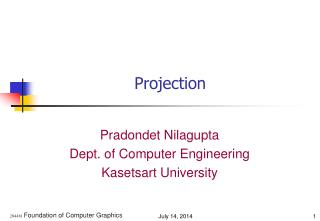DownloadDownload PresentationProjection

# Projection

Download Presentation## Projection

- - - - - - - - - - - - - - - - - - - - - - - - - - - E N D - - - - - - - - - - - - - - - - - - - - - - - - - - -
##### Presentation Transcript

1. Projection Pradondet Nilagupta Dept. of Computer Engineering Kasetsart University 204481 Foundation of Computer Graphics

2. viewport NDC’s World Transformation Projection pipeline This is really called a projection transform Normalize Coordinate Device 204481 Foundation of Computer Graphics

3. Definition (1/2) • Transform points in a coordinate system of dimension n into points in a coordinate system of dimension less than n • The projection is defined by projectors: lines that start from the centre of projection, pass through points on the 3D object and then fall onto the projection plane 204481 Foundation of Computer Graphics

4. Definition (2/2) • Center of projection (CoP): the position of the eye or camera with respect to which the projection is performed (also eyepoint, camera point, projection reference point) • Projection plane: in a 3D->2D projection, the plane to which the projection is performed (also viewplane) 204481 Foundation of Computer Graphics

5. Planar Projections Perspective: Distance to CoP is finite Parallel: Distance to CoP is infinite 204481 Foundation of Computer Graphics

6. Planar Geometry Projection Parallel Perspective Orthographic Oblique One-point Two-point Top (plan) Cabinet Three-point Front elevation Cavalier Other Side elevation Isometric Other Projections 204481 Foundation of Computer Graphics

7. Parallel Projections (1/2) • Orthographic: Direction of projection is orthogonal to the projection plane • There are several types of `axonometric' or parallel projections commonly in use (not orthographic). • Trimetric. Here the coordinate axes remain orthogonal when projected. • Dimetric. Two of the three axes are equally foreshortened when projected. • Isometric. All three axes are equally foreshortened when projected. 204481 Foundation of Computer Graphics

8. Parallel Projections (2/2) • Oblique: Direction of projection is not orthogonal to the projection plane; projection plane is normal to a principal axis • Cavalier: 45° angle with the projection plane • Cabinet: 63.4° angle with the projection plane • All components of the object appear in the 2D image exactly the same size as they do on the original 3D object. • Useful for CAD, but not for visual realism 204481 Foundation of Computer Graphics

9. A Section AA Engineering Drawing 204481 Foundation of Computer Graphics

10. side elevation view Front elevation view Simple viewing: parallel projection • Orthographic • preserves all dimensions • Oblique • preserves some dimensions 204481 Foundation of Computer Graphics

11. z O y x Example of Orthographic Projection on to plane z=0 204481 Foundation of Computer Graphics

12. Isometric Projection (1/2) • There are three stages in this projection. • Rotate through angle A about y axis. • Rotate through angle B about x axis. • Project onto plane z=0. cosA 0 sinA 0 sinBsinA cosB -sinBcosA 0 0 0 0 0 0 0 0 1 204481 Foundation of Computer Graphics

13. Isometric Projection (2/2) Matrix of Isometric Projection 0.7071 0.0 0.7071 0.0 0.4082 0.8166 -0.4082 0.0 0.0 0.0 0.0 0.0 0.0 0.0 0.0 1.0 204481 Foundation of Computer Graphics

14. Example: Isometric View 204481 Foundation of Computer Graphics

15. OpenGL Parallel View glOrtho(left, right, bottom, top, near, far); 204481 Foundation of Computer Graphics

16. z O y x E Perspective Projections Projection on to z=0 • Perspective projections are often considered “more realistic”. • The size of the projected object depends on its distance from the viewpoint. • Lines are projected from viewpoint E through vertex and onto plane. 204481 Foundation of Computer Graphics

17. horizon horizon Perspective Projections • One-point: • One principal axis cut by projection plane • One axis vanishing point • Two-point: • Two principal axes cut by projection plane • Two axis vanishing points • Three-point: • Three principal axes cut by projection plane • Three axis vanishing points 204481 Foundation of Computer Graphics

18. (x, y, z) +Z +Z (xproj, yproj, 0) -Z (0, 0, -d) -Z One point Projection • Center of Projection at the origin with viewplane parallel to the x-y plane a distance d from the origin. • Center of Projection on the negative z-axis with viewplane in the x-y plane. 204481 Foundation of Computer Graphics

19. Matrix for one-Point Projection Center of Projection on the negative z-axis with viewplane in the x-y plane. Center of Projection at the origin with viewplane 204481 Foundation of Computer Graphics

20. Example of Perspective Projection Viewing frustum looks like a truncated Egyptian pyramid 204481 Foundation of Computer Graphics

21. Defining Perspective Projections • glFrustum( l, r, b, t, n, f ); • frustum not necessarily aligned down line of sight • good for simulating peripheral vision • gluPerspective(fovy,aspect,n,f ); • frustum centered down line of sight • more general form • reasonable values: • aspect should match aspect ratio of viewport 204481 Foundation of Computer Graphics

22. Projections in OpenGL Objects not in the view volume are clipped 204481 Foundation of Computer Graphics

23. Perspective Projection • Nonuniform foreshortening – images of objects farther from the center of projection are reduced in size • We apply a 4 x 4 projection matrix after the model-view matrix 204481 Foundation of Computer Graphics

24. OpenGL for Perspective Projection • glFrustum( l, r, b, t, n, f ); • gluPerspective( fovy, aspect, n, f ); • then use glFrustum() 204481 Foundation of Computer Graphics

25. OpenGL Perspective (1/2) glMatrixMode(GL_PROJECTION); glLoadIdentity( ); glFrustum(left, right, bottom, top, near, far); 204481 Foundation of Computer Graphics

26. OpenGL Perspective (2/2) gluPerspective(fovy, aspect, near, far); fov is the angle between the top and bottom planes 204481 Foundation of Computer Graphics

27. Standard Viewing Volume 204481 Foundation of Computer Graphics